# Tips for Physics Practical Exams

The following guidelines come as a result of doing all the London Examinations (now Edexcel) PH10 (International) practical exams from 1995 to 2000 and reading the mark schemes for them. They are designed to help you as much as possible to give the points the examiners want. If you get a chance, it's a good idea to read through a mark scheme and the examiner's comments. Also, there is no substitute for practice: do as many past exams as you can!

## Oscillations

• Perform 3 sets of measurements for each different oscillation, (you will get more marks if you do 2 sets of repeats).
• Measure at least 30 periods in total.
• Preferably make each measurement 20 periods long.
• Precaution: minimise oscillations in any other plane other than the one being observed ("Careful release to avoid unwanted modes of oscillation"). Explain how you did this.
• Always write times to two d.p., never to the nearest second.
• Precaution: do small amplitude oscillations
• Precaution: say that you did several periods at once to minimise reaction time errors.
• Precaution: use a fiducial marker at the centre of the oscillation.

## Moments

• Precaution: balance the ruler being used first.
• Use distances from the pivot of greater than 25 cm (250 mm).
• Weigh the unknown and known masses in your hands before using the ruler, and place the lighter mass as far from the pivot as possible.
• Measure distances from the pivot to the centre of mass of the object.
• All measurements should be to 1 mm accuracy.
• To make sure the rule is balanced, pull each end down. If it comes up again then the rule must have equal moments acting on it on either end.

## Density Measurements

• When using a micrometer, one full rotation of the barrel is 0.5 mm (50 on the barrel scale).
• The micrometer reads to 0.01 mm accuracy.
• Vernier callipers can read to 0.05 mm accuracy. However, it is advisable to quote the figure to 0.1 mm accuracy, as this will give a larger uncertainty, which will make comparisons in later parts of the question easier.
• If measuring a very small dimension, measure several "thicknesses" of it. Remember to divide by the number of thicknesses after the measurement!
• Precaution: check the zero error on the micrometer and/or Vernier callipers. Wipe the jaws of the micrometer to remove grease.
• Give answers to 2 or 3 s.f. : it is meaningless to write, for example, "density is 7785.654 kg m-3"!
• Always take at least 3 readings for each measurement, and take an average.
• When measuring string diameters or foil thicknesses (or similar), use a minimum of 10 thicknesses.

## Miscellaneous

• With a measurement that varies substantially each time you test it, take at least 4 readings. An example is sand falling from a funnel.
• For Experiment C, 8 readings are normally sufficient (sometimes even 6). Extra time at the end can be used for writing up the other experiments or planning them.
• Show clearly on diagrams your measurements, e.g. to the centres of mass of objects when doing a moments experiment.
• In any experiment that involves loading and unloading, state that you checked the original height on unloading each time.
• In any experiment involving, e.g. a funnel and sand, state that you kept the funnel vertical.
• Precaution: with many experiments, you can state that you did something to avoid parallax error.
• When measuring angles, measure from the underside of, e.g., the board you are measuring the angle of, compared to the horizontal bench.
• To measure a height with a ruler and a set square, hold the ruler vertically using the set square against the bench and the flat side of the ruler.
• Show any angles measured on diagrams, including ones of forces.

## Uncertainties

• % Uncertainty =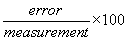• When multiplying or dividing quantities, add their % uncertainties together.
• When adding or subtracting quantities, add their absolute errors together, then divide by the result of the addition/subtraction of the measurement, e.g. for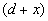, where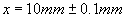, and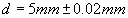, the absolute errors added = 0.102 mm. Therefore the % uncertainty is: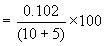• If you have to calculate the error in, e.g.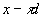, the absolute error in d must be multiplied by pi and then added to the absolute error in x. The percentage uncertainty is this total error divided by the calculatedand the result multiplied by 100.
• If a measurement is to be raised to a power, then multiply the % uncertainty in the measurement by the power to get the % uncertainty in the overall term.
• If two values, for say, a density are available, calculate the % difference between them. If a value is given by the examiner, then use this as the "correct" value, and calculate the % difference the following way: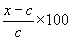where x is your measured value, and c is the examiner's value. If you have obtained two values, then the expression changes: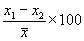where x1 and x2 are your measured values, and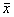is the median of the two, (not necessarily the mean!).
• Compare the % difference with your % uncertainty. Any relationship suggested, such as that the two densities should be equal, can be considered correct if your % difference is less than your % uncertainty. This will gain you marks!
• If your % uncertainties look small, check that you have multiplied by 100!

## Electrical Experiments

• With capacitor discharges, either take readings every 5 seconds for the first part of the discharge, or I think that every 10 seconds is sufficient.
• If a range is specified over which you should take measurements, do not exceed it: you will be penalised.
• With an analogue ammeter, use the top scale. This reads (generally), from 20, to 0, to 10. These are in fact divisions of 10 m A, and the meter actually reads from -20 to 100 m A. If you are out by a factor of 10, (e.g. you get a calculated cell voltage of 0.15 V), check that you have read the meter correctly. Always remember that the polarity on the meter must be correct.
• Any small discrepancy in your results can be explained by "electrical resistance at the contacts in the circuit".
• With most electrical experiment where a curve will be obtained (e.g. the V/I characteristic of a diode), 9 points on a smooth curve are sufficient.

## Graphs

• With any graph, a minimum of 6 to 8 points are needed, and you must have at least 4 points on a curve.
• When measuring the gradient of a graph, carry your tangent on to the sides of the graph paper, however big your graph. The triangle you use should be greater than 10 cm in length and height, although in some mark schemes 100 cm2 is fine.
• Your graph does not have to go through the origin. If the data does not indicate that it does so, do not force it to. Comment on the fact that there must have been a systematic error.
• When choosing values to read of a graph, it is better to take them from the middle part of the curve, as this is where you will have more points per change in y co-ordinate.
• When describing your "plan" in Experiment C, state that the graph you plot will be a straight line through the origin (if this is the case!), of gradient = to an expression which will help you confirm the relationship suggested by the examiner.
• Turning points on graphs require at least 4 points.
• If points near the origin deviate substantially from your line of best fit, point out that for small measurements there is a greater uncertainty.

## Experiments Involving Temperature

• Readings should be accurate to fractions of a degree.
• Stir any liquid being heated.
• Insulate the apparatus if possible.
• The thermometer should not be touching the sides of the container it is in, and should be in the middle of the liquid you are measuring the temperature of.
• Precaution: read the thermometer at eye level to avoid parallax error.
• The bulb of the thermometer should be completely submerged.
• Comment on the result obtained being the right order of magnitude.

Finally, always read the question carefully!